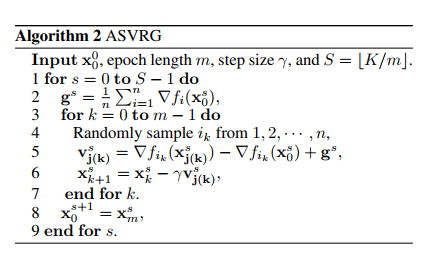# Parallel Asynchronous Stochastic Variance Reduction for Nonconvex Optimization### Abstract

Nowadays, asynchronous parallel algorithms have received much attention in the optimization field due to the crucial demands for modern large-scale optimization problems. However, most asynchronous algorithms focus on convex problems. Analysis on nonconvex problems is lacking. For the Asynchronous Stochastic Descent (ASGD) algorithm, the best result from (Lian et al., 2015) can only achieve an asymptotic O(1/eps^2) rate (convergence to the stationary points) on nonconvex problems. In this paper, we study Stochastic Variance Reduced Gradient (SVRG) in the asynchronous setting. We propose the Asynchronous Stochastic Variance Reduced Gradient (ASVRG) algorithm for nonconvex finite-sum problems. We develop two schemes for ASVRG, depending on whether the parameters are updated as an atom or not. We prove that both of the two schemes can achieve linear speed up (a non-asymptotic O(n^{2/3}/eps) rate to the stationary points) for nonconvex problems when the delay parameter τ <= n^{1/3} , where n is the number of training samples. We also establish a non-asymptotic O(n^{2/3}τ^{1/3}/eps) rate (convergence to the stationary points) for our algorithm without assumptions on τ . This further demonstrates that even with asynchronous updating, SVRG has less number of Incremental First-order Oracles (IFOs) compared with Stochastic Gradient Descent and Gradient Descent. We also conduct experiments on a shared memory multi-core system to demonstrate the efficiency of our algorithm.

Type
Publication
AAAI Conference on Artificial Intelligence